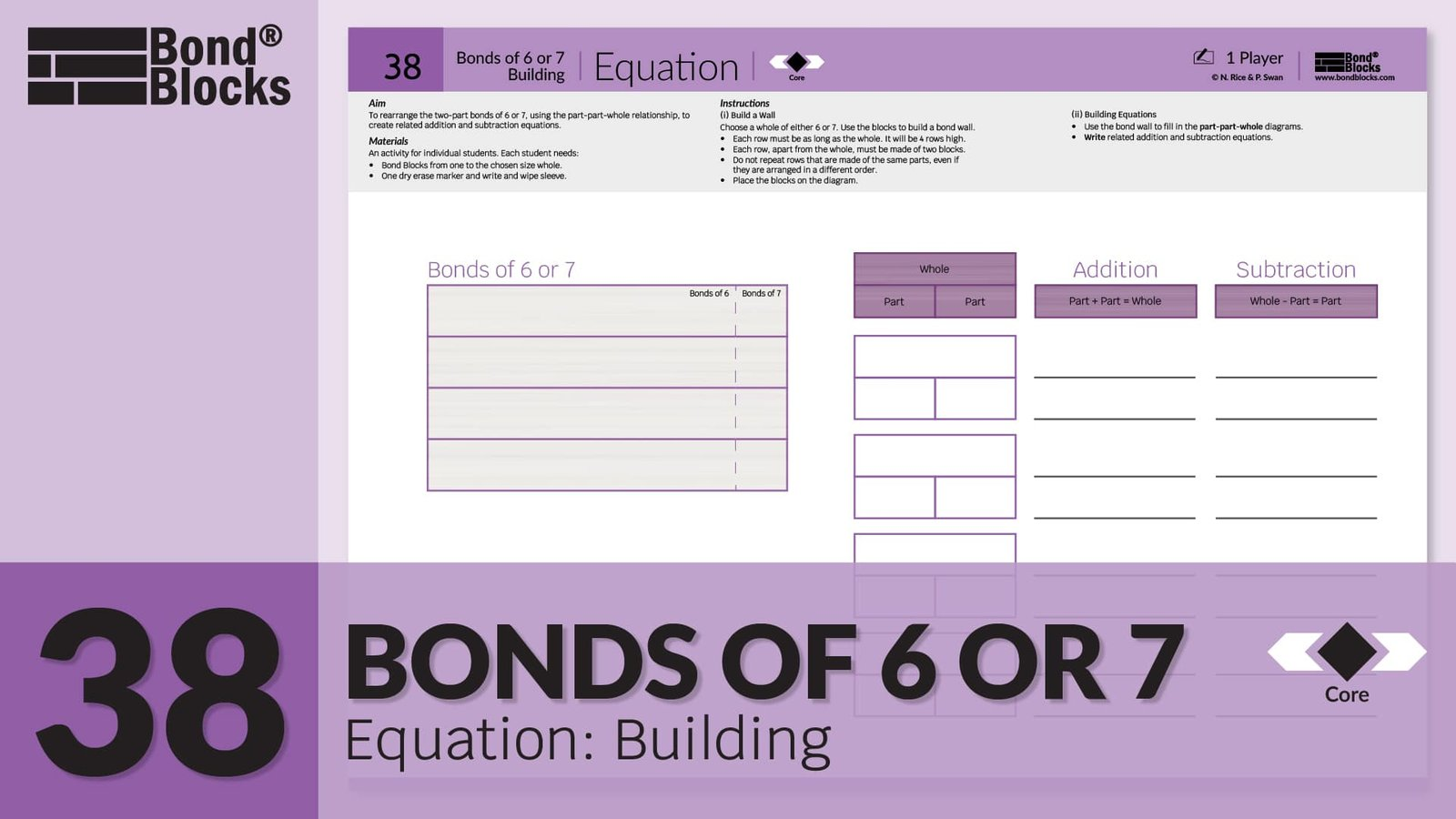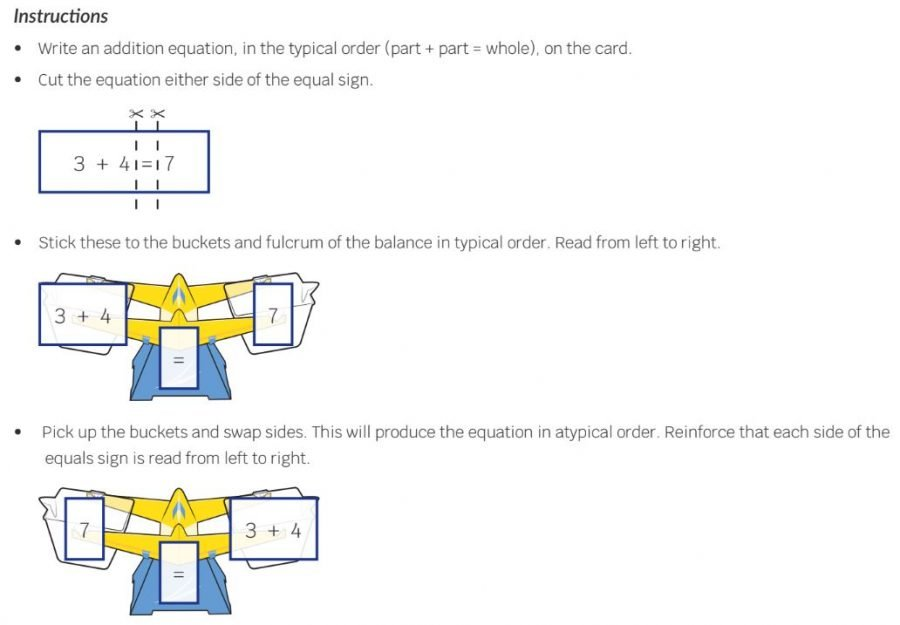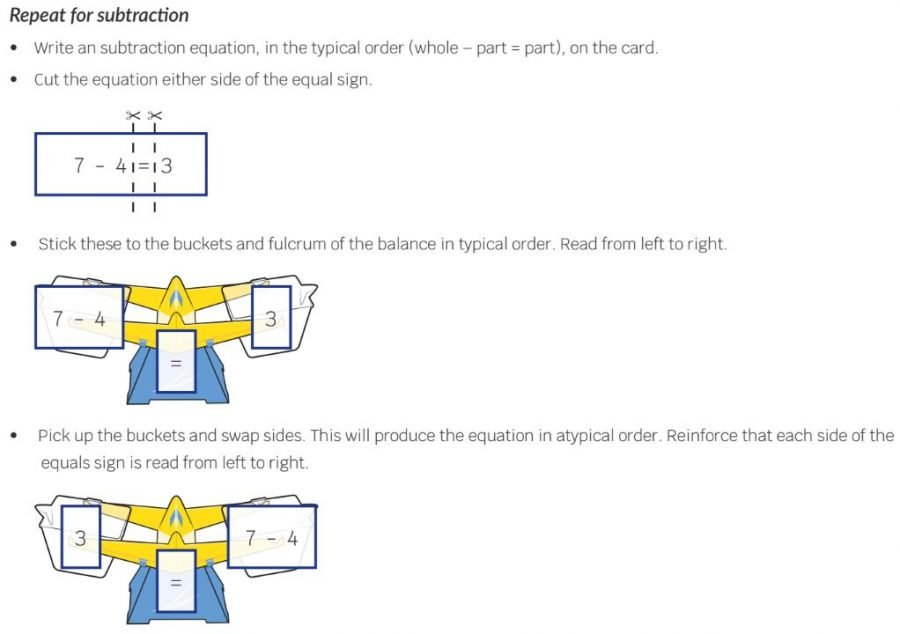## 38) Equation

Bonds of 6, 7, 8, 9: Building### Mathematics

Develop the concept of related addition and subtraction equations, rearranged using the part-part-whole relationship, for two-part bonds of 6, 7, 8 and 9.

### Language

• part-part-whole: where the two parts are a bond of the whole number.
• equals, is equal to
• subtraction, subtract, take away

### Core Activity Support MaterialsClick to open in a new tab.## Differentiation

### A little easier

##### Equation building

The teacher models Activity 38 Equation Building  for Bonds of 6, linking the Bond Blocks, part-part-whole diagram and written equations. This is demonstrated for Bonds of 6 in the video at the top of this page. Leave the worked example on the activity board out for the student to use. The student uses this to complete the same activity for two-part Bonds of 7.

Repeat this. The teacher models Activity 38 Equation Building for Bonds of 8. This is demonstrated in the video below. The student uses this worked example to complete the same activity for two-part bonds Bonds of 9.

### A little harder

##### Atypical arrangement

Addition and subtraction equations don’t have to be written as

Part + Part = Whole

Whole – Part = Part

An alternative arrangement is

Whole = Part + Part

Part = Whole – Part